# Newtons Laws of Motion Newtons First Law of

• Slides: 52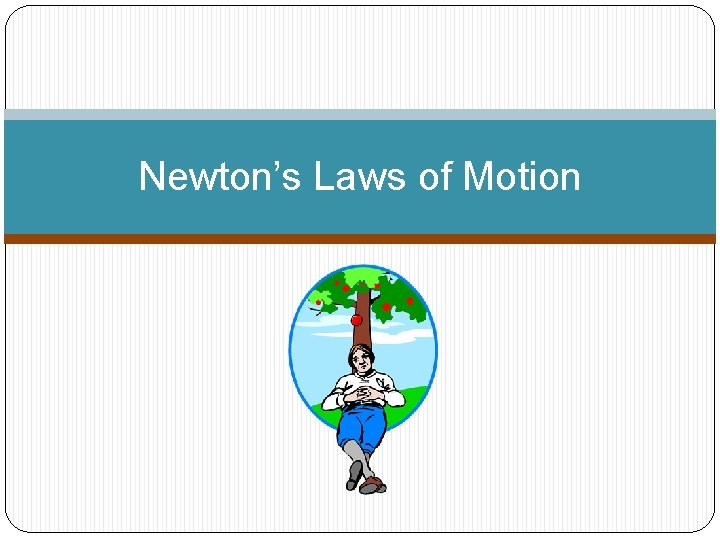Newton’s Laws of Motion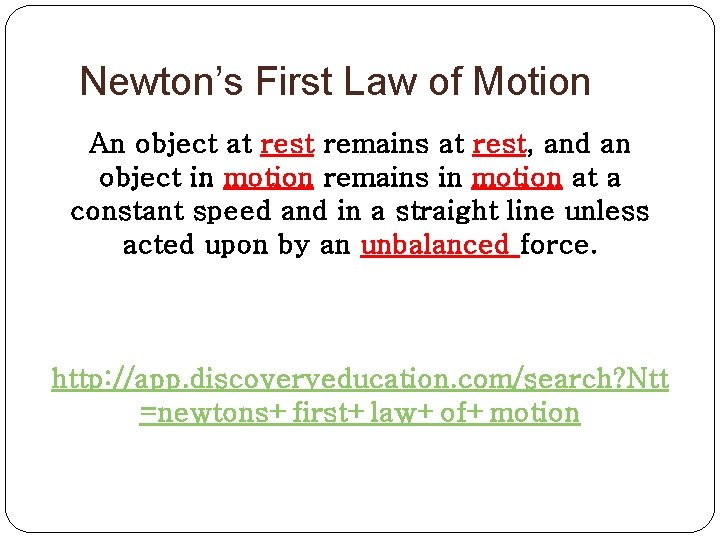Newton’s First Law of Motion An object at rest remains at rest, and an object in motion remains in motion at a constant speed and in a straight line unless acted upon by an unbalanced force. http: //app. discoveryeducation. com/search? Ntt =newtons+first+law+of+motion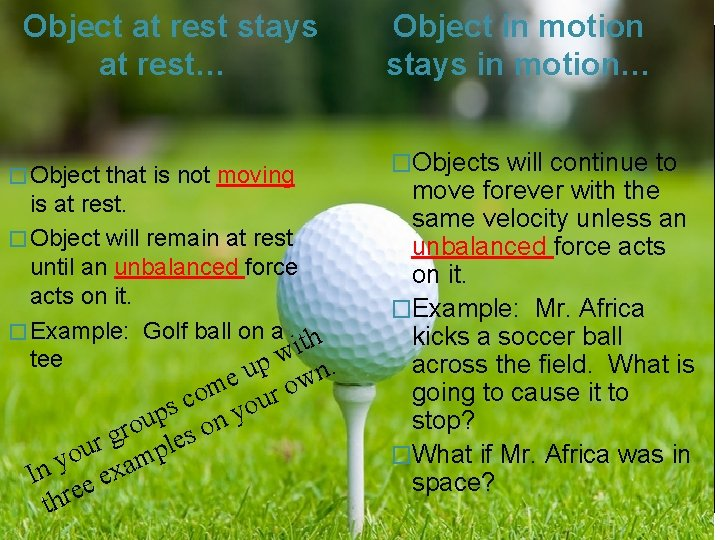Object at rest stays at rest… � Object that is not moving is at rest. � Object will remain at rest until an unbalanced force acts on it. � Example: Golf ball on a h t i w tee up . n e w o m o r c you s p u n o o r g s e r l u p o m y a n x I e e thre Object in motion stays in motion… �Objects will continue to move forever with the same velocity unless an unbalanced force acts on it. �Example: Mr. Africa kicks a soccer ball across the field. What is going to cause it to stop? �What if Mr. Africa was in space?Newton’s First Law is sometimes called the Law of Inertia �INERTIA is tendency of all objects to resist any change in motion. �Example: Mrs. Wulf is in a car crash and she is not wearing her seat belt. What is going to happen? https: //www. youtube. com/watch? v=d 7 i. YZPp 2 z. YY �Mass is a measure of inertia �An object that has a small mass has less inertia than an object that has a large mass. �Example : It’s easier to throw a softball than a bowling ball.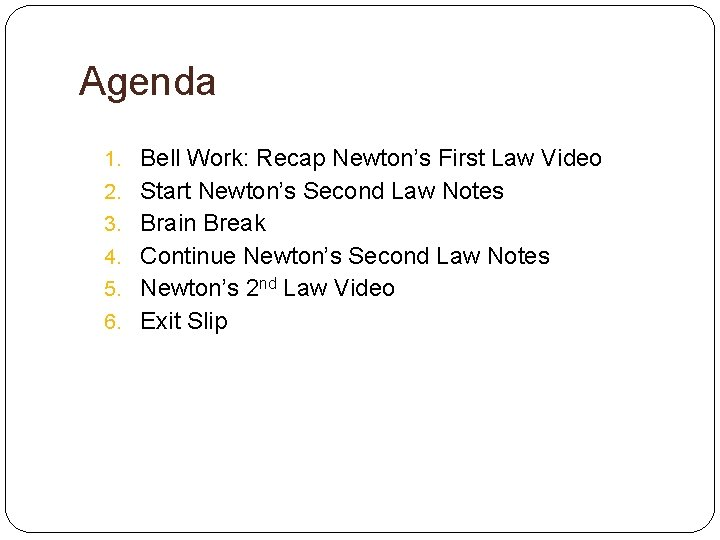Agenda 1. Bell Work: Recap Newton’s First Law Video 2. Start Newton’s Second Law Notes 3. Brain Break 4. Continue Newton’s Second Law Notes 5. Newton’s 2 nd Law Video 6. Exit Slip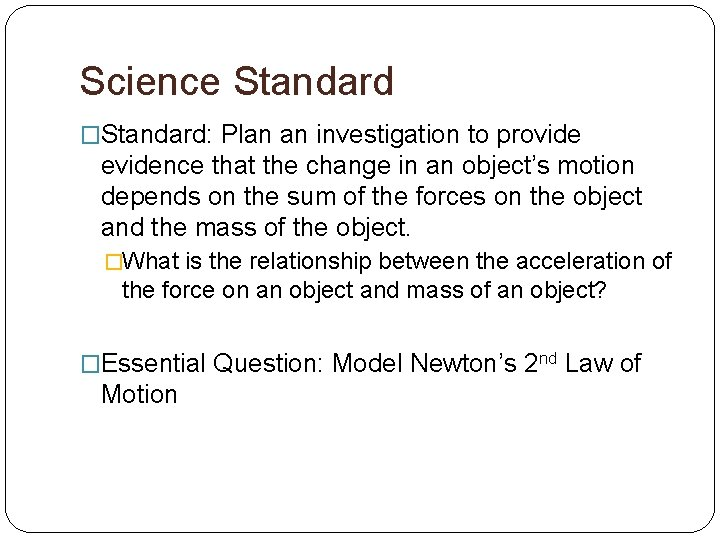Science Standard �Standard: Plan an investigation to provide evidence that the change in an object’s motion depends on the sum of the forces on the object and the mass of the object. �What is the relationship between the acceleration of the force on an object and mass of an object? �Essential Question: Model Newton’s 2 nd Law of MotionNewton’s Second Law of Motion Definition: The acceleration of an object depends on the mass of the object and the amount of force applied.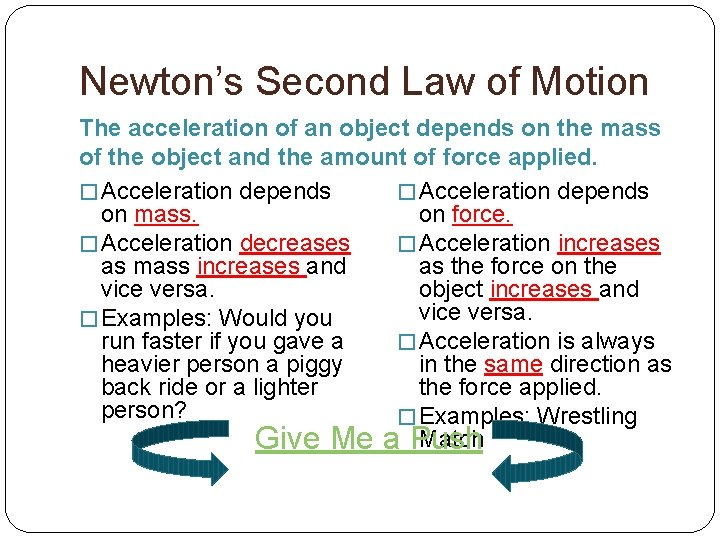Newton’s Second Law of Motion The acceleration of an object depends on the mass of the object and the amount of force applied. � Acceleration depends on mass. on force. � Acceleration decreases � Acceleration increases as mass increases and as the force on the vice versa. object increases and vice versa. � Examples: Would you run faster if you gave a � Acceleration is always heavier person a piggy in the same direction as back ride or a lighter the force applied. person? � Examples: Wrestling Match Give Me a Push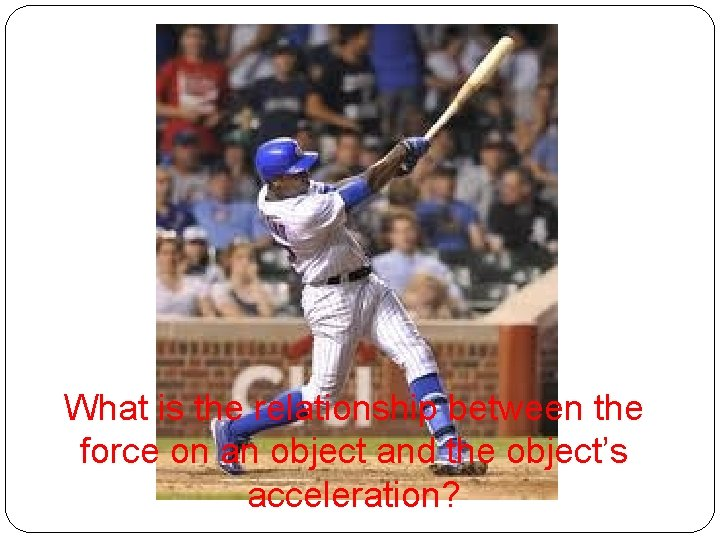What is the relationship between the force on an object and the object’s acceleration?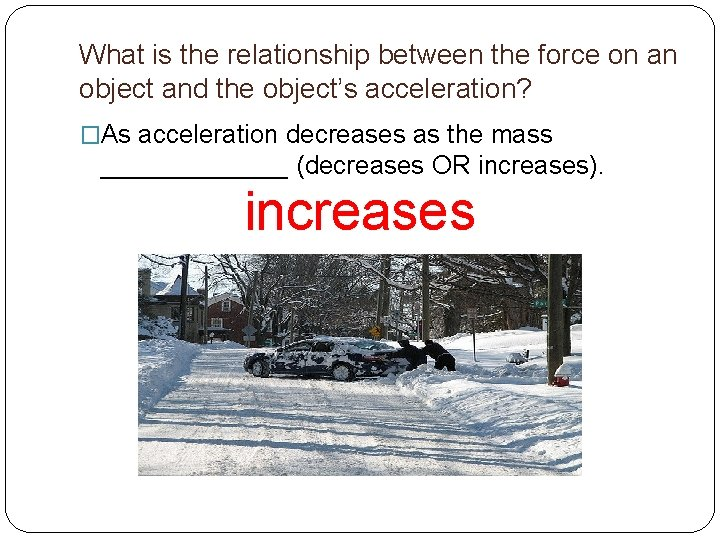What is the relationship between the force on an object and the object’s acceleration? �As acceleration decreases as the mass _______ (decreases OR increases). increases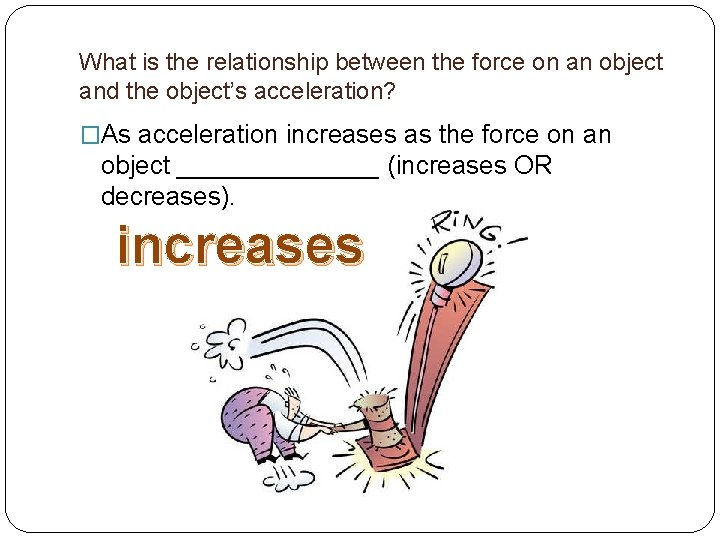What is the relationship between the force on an object and the object’s acceleration? �As acceleration increases as the force on an object _______ (increases OR decreases). increasesBrain Break Extreme Rock, Paper, Scissors Game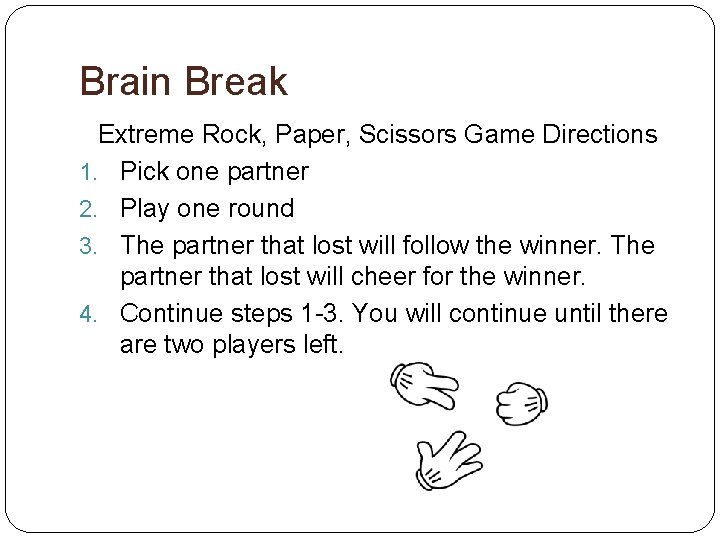Brain Break Extreme Rock, Paper, Scissors Game Directions 1. Pick one partner 2. Play one round 3. The partner that lost will follow the winner. The partner that lost will cheer for the winner. 4. Continue steps 1 -3. You will continue until there are two players left.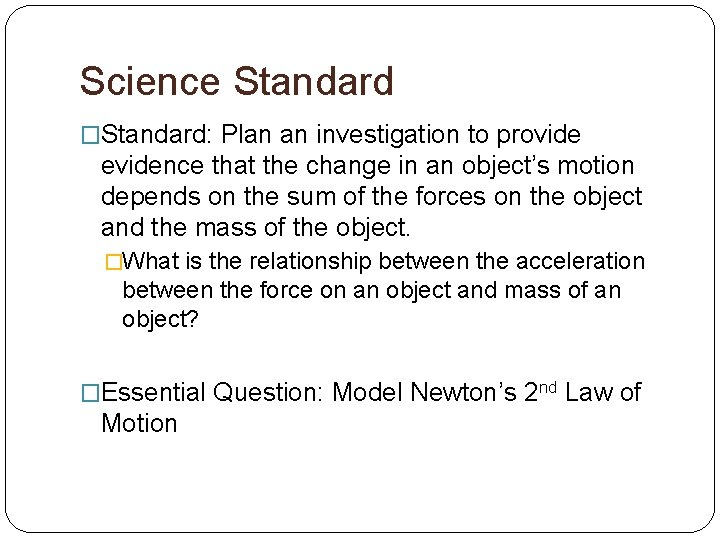Science Standard �Standard: Plan an investigation to provide evidence that the change in an object’s motion depends on the sum of the forces on the object and the mass of the object. �What is the relationship between the acceleration between the force on an object and mass of an object? �Essential Question: Model Newton’s 2 nd Law of Motion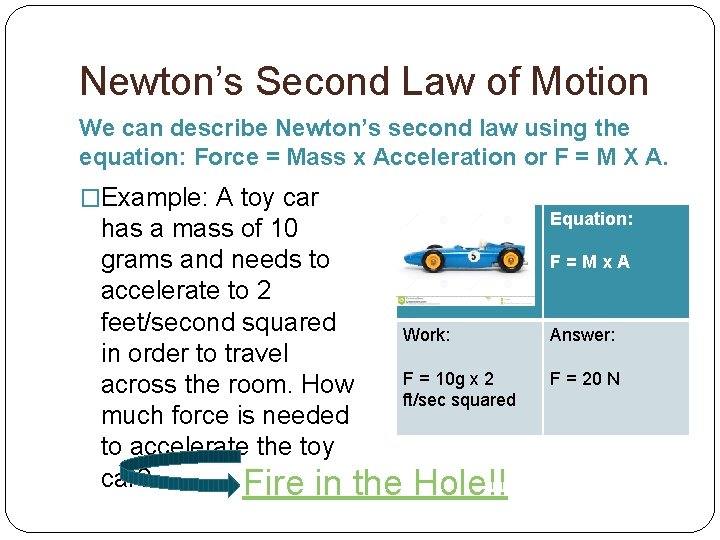Newton’s Second Law of Motion We can describe Newton’s second law using the equation: Force = Mass x Acceleration or F = M X A. �Example: A toy car has a mass of 10 grams and needs to accelerate to 2 feet/second squared Work: in order to travel F = 10 g x 2 across the room. How ft/sec squared much force is needed to accelerate the toy car? Fire in the Hole!! Equation: F=Mx. A Answer: F = 20 NNewton’s Second Law of Motion Now your Try: �Example: A little boy needs a 5 gram weight to accelerate 10 inches per second squared in order to win a prize a the carnival. How much force does he need in order to win the prize? Baby Thor's Hammer Equation: F=Mx. A Work: Answer: F =5 g x 10 in/sec F = 50 N squaredExit Slip Get out a half sheet of paper. Make sure you write your name at the top. 1. As acceleration decreases as the mass ______ (increases or decreases). 2. As acceleration increases the force on the object _____ (increases or decreases). 3. From a scale 1 -4, how do you feel about Newton’s Second Law? 1: I don’t understand this yet. 2. I need more practice. 3. I understand can do it myself. 4. I can do this and explain it to someone else.Friday Agenda �Bell work: Answer the Unit Objective C question, Model Newton’s 2 nd Law of Motion 1. Write it In your own words. 2. Write What I’ve Learned �Work with an assigned partner on the Forces and Claims Practice. �Start Newton’s Third Law of Motion Notes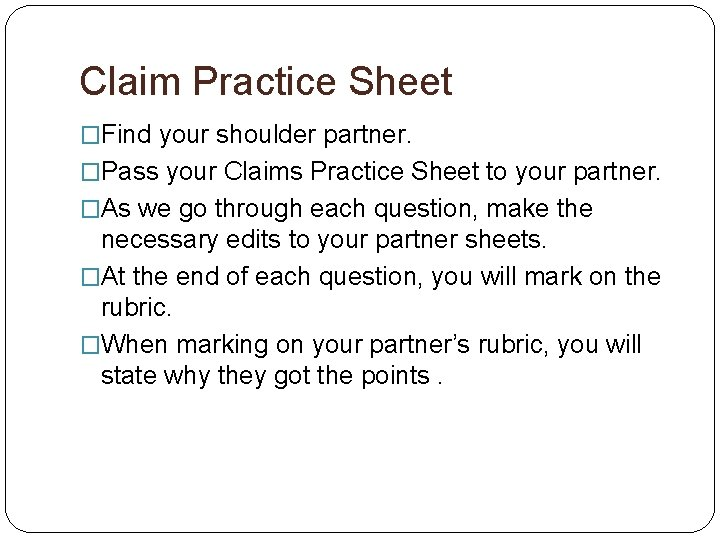Claim Practice Sheet �Find your shoulder partner. �Pass your Claims Practice Sheet to your partner. �As we go through each question, make the necessary edits to your partner sheets. �At the end of each question, you will mark on the rubric. �When marking on your partner’s rubric, you will state why they got the points.Science Standard: MS-PS 2 -1 Motion and Stability: Forces and Interactions �Standard: Apply Newton’s Third Law to design a solution to a problem involving the motion of two colliding objects. �Essential Question: Model Newton’s Third Law of Motion. �I know I got it if: You can explain the relationship of acceleration and the mass and the acceleration and force on an object. �I’ll Know I get it if: You agree with the following statement: �For every action force, there is always a reaction force.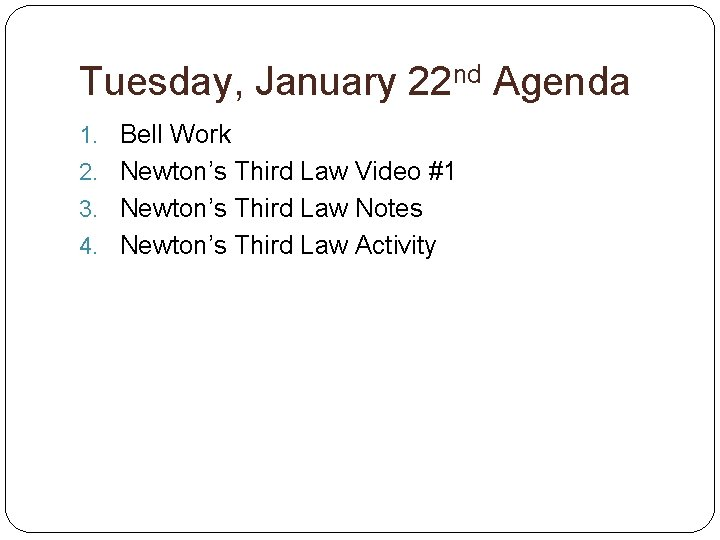Tuesday, January 22 nd Agenda 1. Bell Work 2. Newton’s Third Law Video #1 3. Newton’s Third Law Notes 4. Newton’s Third Law Activity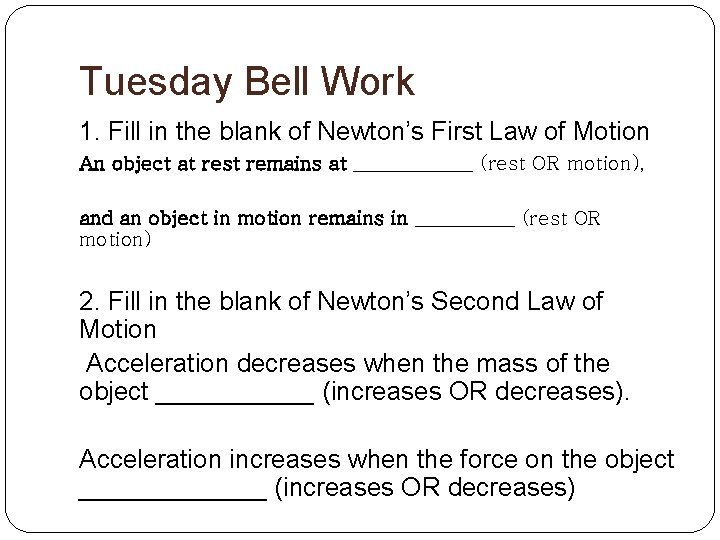Tuesday Bell Work 1. Fill in the blank of Newton’s First Law of Motion An object at rest remains at ______ (rest OR motion), and an object in motion remains in _____ (rest OR motion) 2. Fill in the blank of Newton’s Second Law of Motion Acceleration decreases when the mass of the object ______ (increases OR decreases). Acceleration increases when the force on the object _______ (increases OR decreases)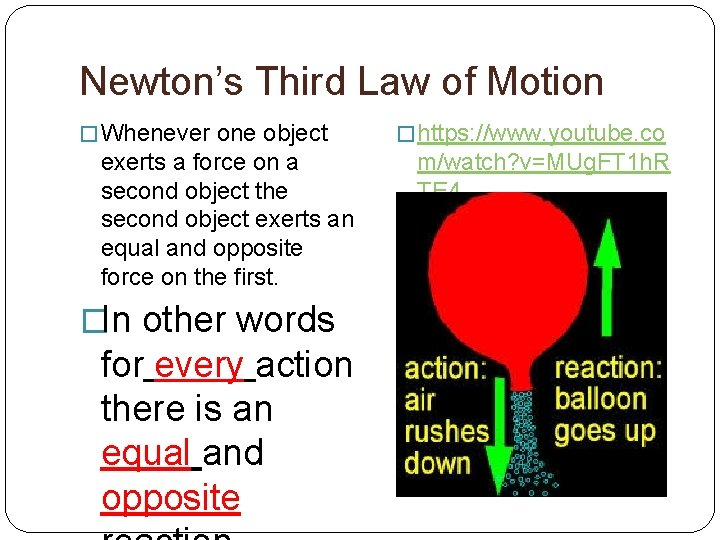Newton’s Third Law of Motion � Whenever one object exerts a force on a second object the second object exerts an equal and opposite force on the first. �In other words for every action there is an equal and opposite � https: //www. youtube. co m/watch? v=MUg. FT 1 h. R TE 4Continued… �All forces act in pairs. �If a force is exerted, another force occurs that is equal in size and opposite in direction. �The way the force pairs interact affects the motion of objects.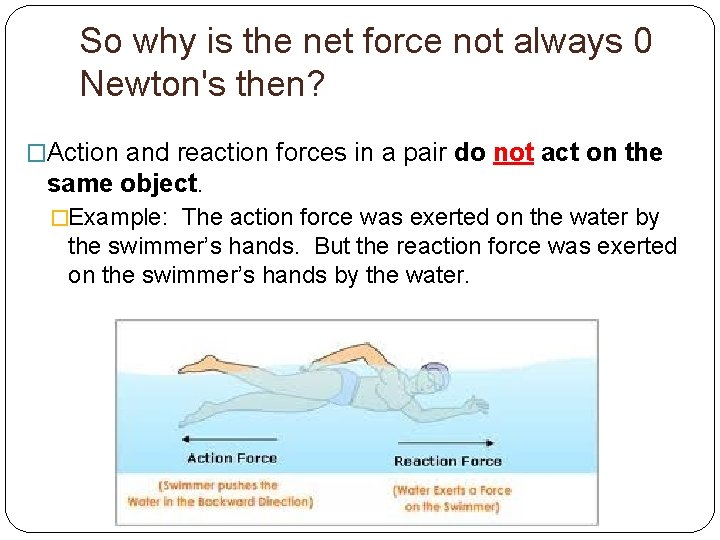So why is the net force not always 0 Newton's then? �Action and reaction forces in a pair do not act on the same object. �Example: The action force was exerted on the water by the swimmer’s hands. But the reaction force was exerted on the swimmer’s hands by the water.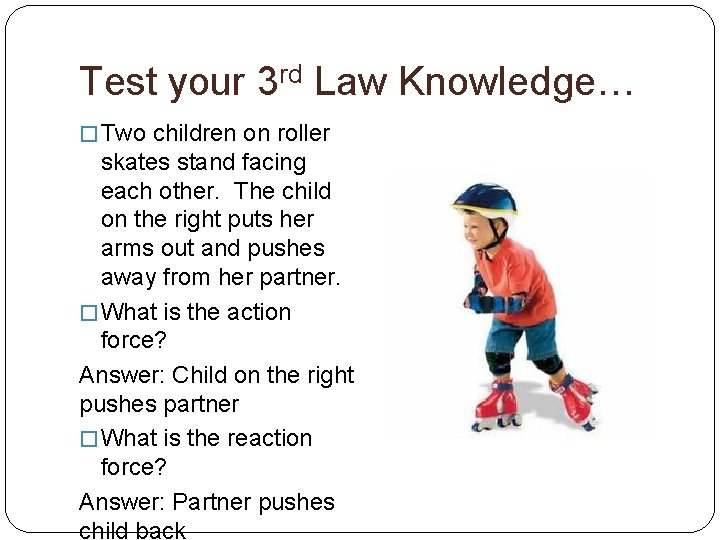Test your 3 rd Law Knowledge… � Two children on roller skates stand facing each other. The child on the right puts her arms out and pushes away from her partner. � What is the action force? Answer: Child on the right pushes partner � What is the reaction force? Answer: Partner pushes child back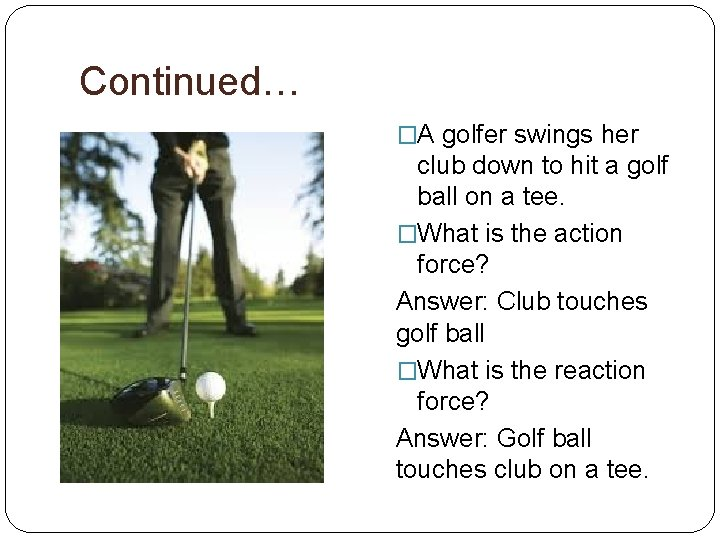Continued… �A golfer swings her club down to hit a golf ball on a tee. �What is the action force? Answer: Club touches golf ball �What is the reaction force? Answer: Golf ball touches club on a tee.Last one! �A frog sits on a lily pad in the middle of a pond. Suddenly it makes a leap pushing off the lily pad. �What is the action force? �What is the reaction force? Action Force: Frog pushes down on lily pad Reaction Force: Lily pad touches frog.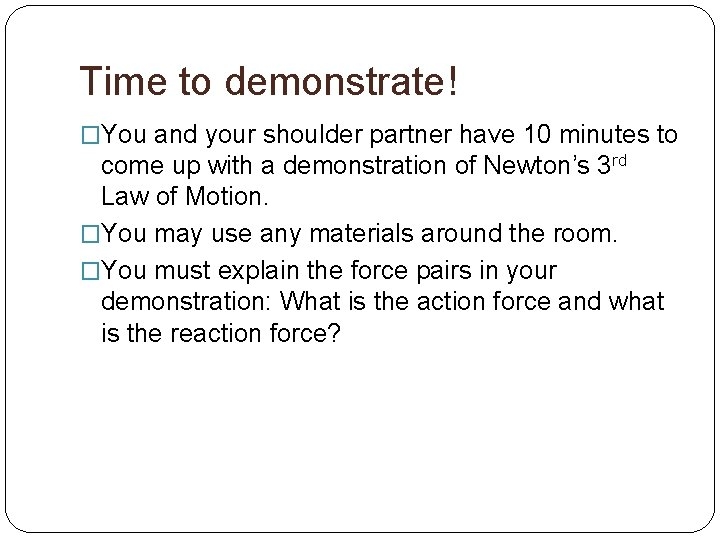Time to demonstrate! �You and your shoulder partner have 10 minutes to come up with a demonstration of Newton’s 3 rd Law of Motion. �You may use any materials around the room. �You must explain the force pairs in your demonstration: What is the action force and what is the reaction force?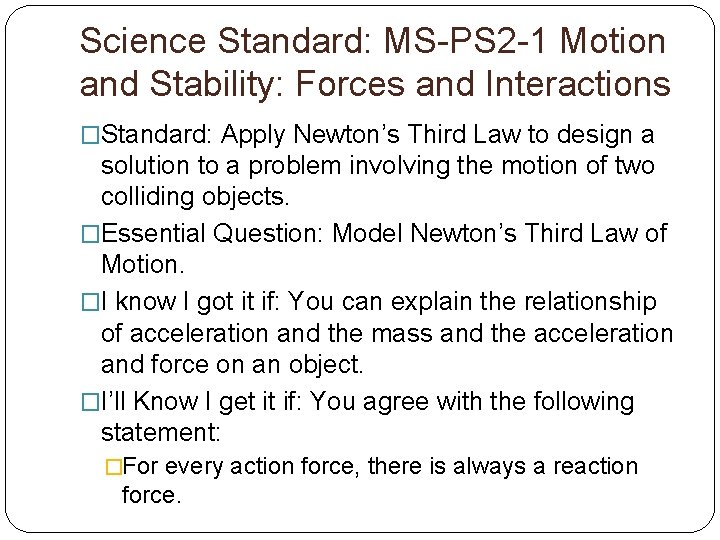Science Standard: MS-PS 2 -1 Motion and Stability: Forces and Interactions �Standard: Apply Newton’s Third Law to design a solution to a problem involving the motion of two colliding objects. �Essential Question: Model Newton’s Third Law of Motion. �I know I got it if: You can explain the relationship of acceleration and the mass and the acceleration and force on an object. �I’ll Know I get it if: You agree with the following statement: �For every action force, there is always a reaction force.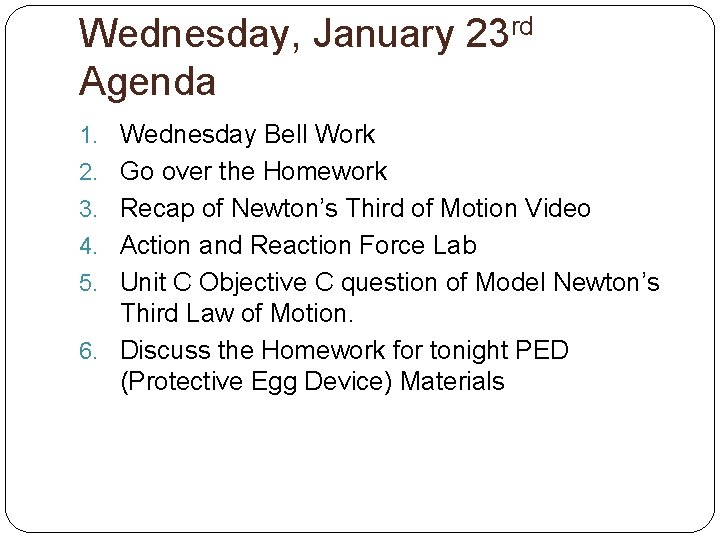Wednesday, January 23 rd Agenda 1. Wednesday Bell Work 2. Go over the Homework 3. Recap of Newton’s Third of Motion Video 4. Action and Reaction Force Lab 5. Unit C Objective C question of Model Newton’s Third Law of Motion. 6. Discuss the Homework for tonight PED (Protective Egg Device) MaterialsWednesday Bell Work 1. For every action force there is a _____ (action OR reaction) force. 2. All forces act in _____ (pairs OR alone) 3. If a force is exerted, another force occurs that is equal in size and opposite in _______(direction OR speed). 4. Rate yourself how you feel 1. I don’t understand. I need help! 2. I feel okay 3. I feel good 4. I feel great! I feel confident teaching another peer.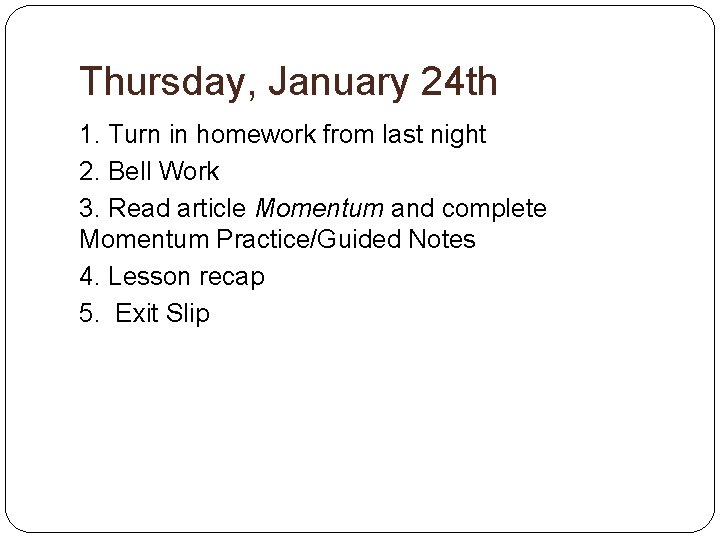Thursday, January 24 th 1. Turn in homework from last night 2. Bell Work 3. Read article Momentum and complete Momentum Practice/Guided Notes 4. Lesson recap 5. Exit SlipThursday Bell Work 1. What do you think the word momentum means? 2. Do you think momentum is related to Newton’s three laws of motion? 3. If , “yes”, explain why you think momentum is related to Newton’s three laws of motion. You can list Newton’s law/s of motion. OR If , “no”, explain why you think momentum is not related to Newton’s three laws of motion. At 8: 03, I will have you discuss your answers with a nearby classmate.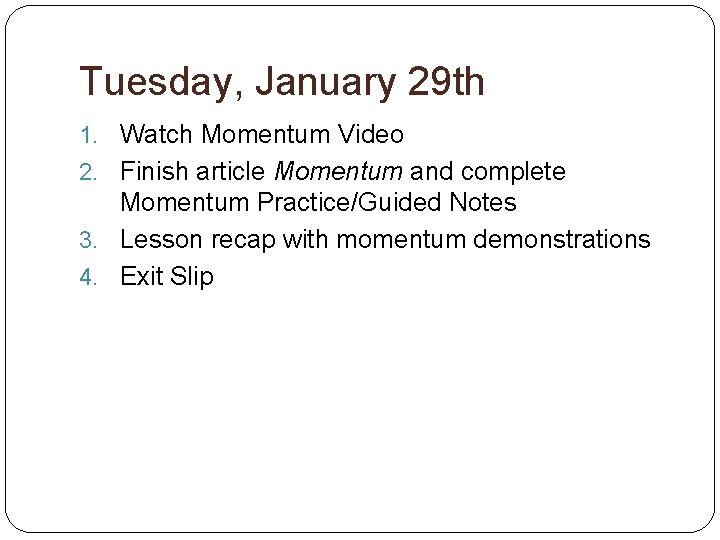Tuesday, January 29 th 1. Watch Momentum Video 2. Finish article Momentum and complete Momentum Practice/Guided Notes 3. Lesson recap with momentum demonstrations 4. Exit Slip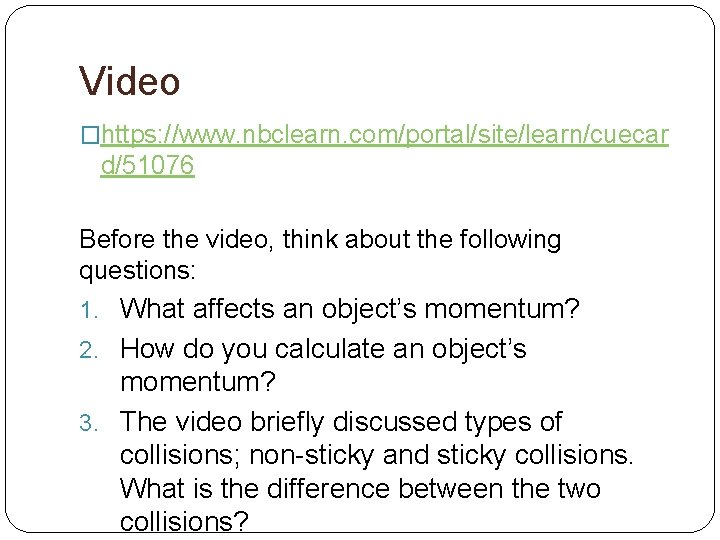Video �https: //www. nbclearn. com/portal/site/learn/cuecar d/51076 Before the video, think about the following questions: 1. What affects an object’s momentum? 2. How do you calculate an object’s momentum? 3. The video briefly discussed types of collisions; non-sticky and sticky collisions. What is the difference between the two collisions?After Video 1. What affects an object’s momentum? Answer: Mass and Velocity 2. How do you calculate an object’s momentum? Answer: Mass x Velocity 3. The video briefly discussed types of collisions; non-sticky and sticky collisions. What is the difference between the two collisions? Think: What is the difference a sticky candy bar and a candy bar that is not sticky?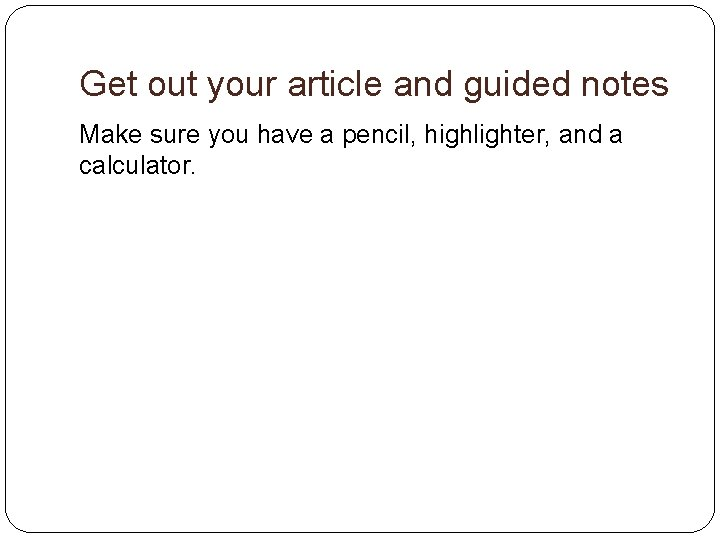Get out your article and guided notes Make sure you have a pencil, highlighter, and a calculator.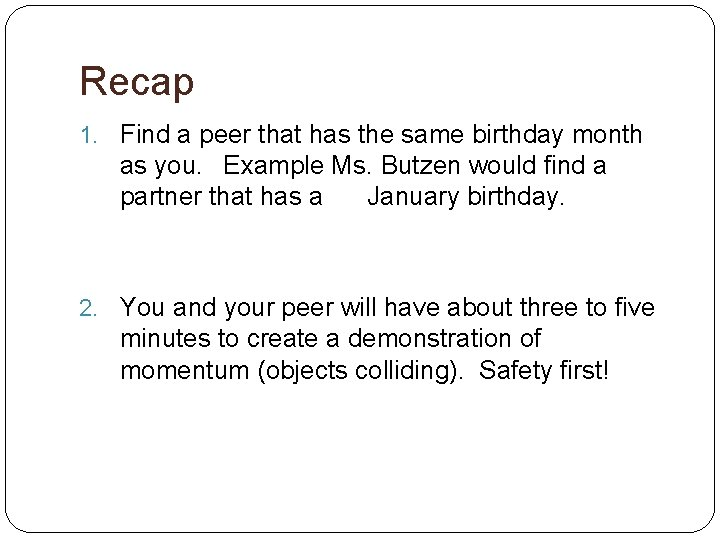Recap 1. Find a peer that has the same birthday month as you. Example Ms. Butzen would find a partner that has a January birthday. 2. You and your peer will have about three to five minutes to create a demonstration of momentum (objects colliding). Safety first!EXIT SLIP �Try your best. �Once you are done, pick the exit slip in the orange turn in bin.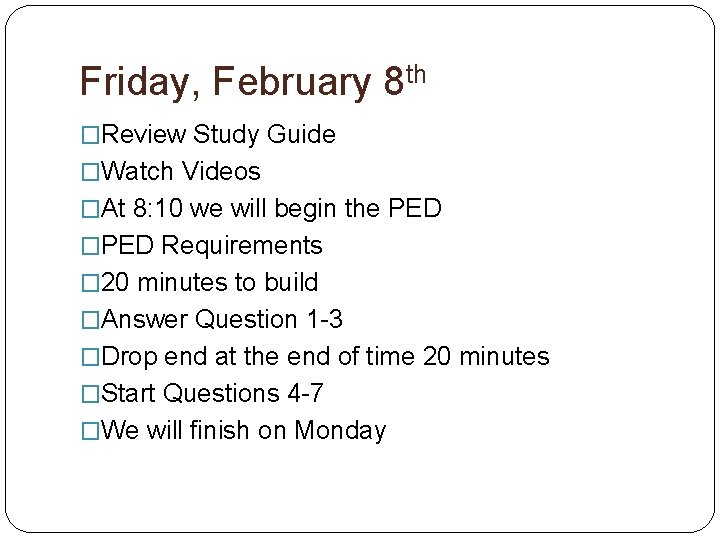Friday, February 8 th �Review Study Guide �Watch Videos �At 8: 10 we will begin the PED �PED Requirements � 20 minutes to build �Answer Question 1 -3 �Drop end at the end of time 20 minutes �Start Questions 4 -7 �We will finish on Monday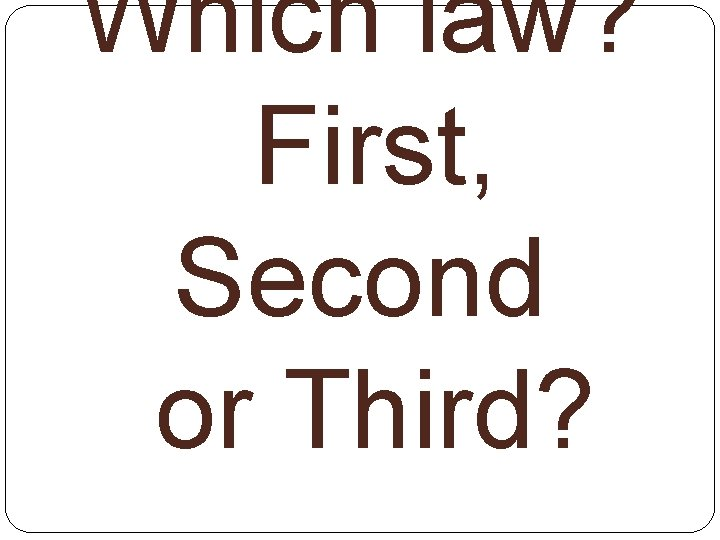Which law? First, Second or Third?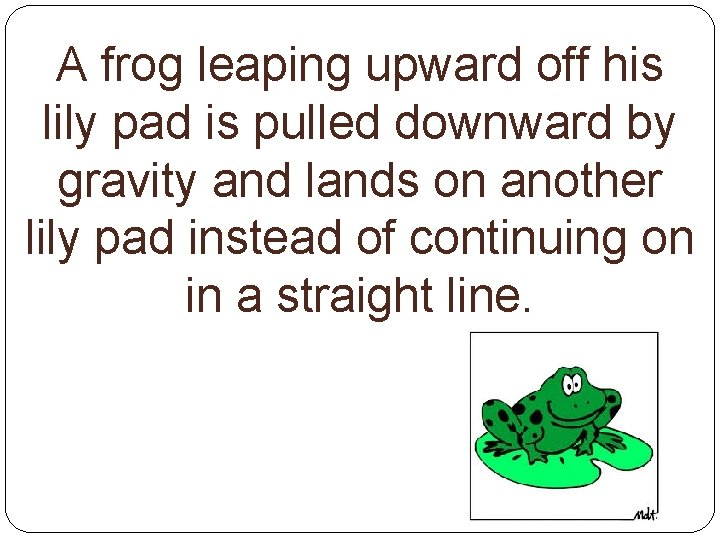A frog leaping upward off his lily pad is pulled downward by gravity and lands on another lily pad instead of continuing on in a straight line.As the fuel in a rocket ignites, the force of the gas expansion and explosion pushes out the back of the rocket and pushes the rocket forward.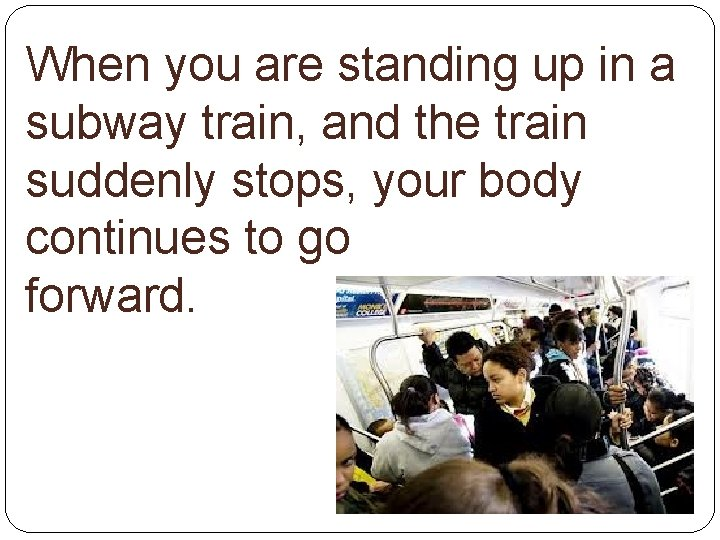When you are standing up in a subway train, and the train suddenly stops, your body continues to go forward.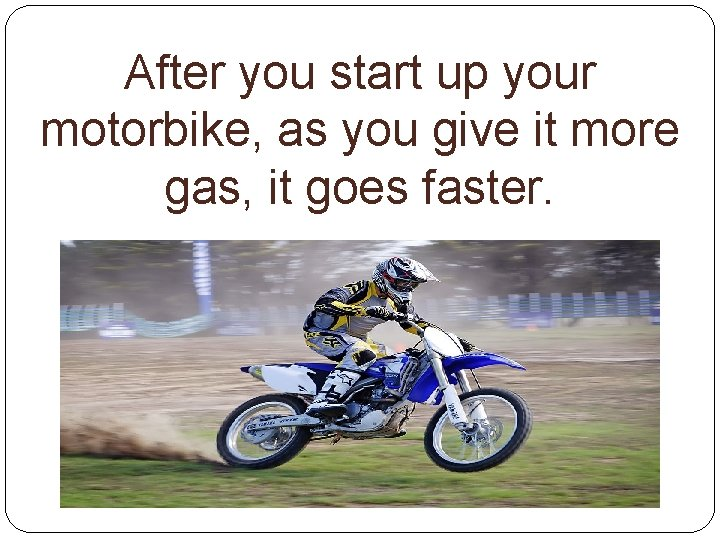After you start up your motorbike, as you give it more gas, it goes faster.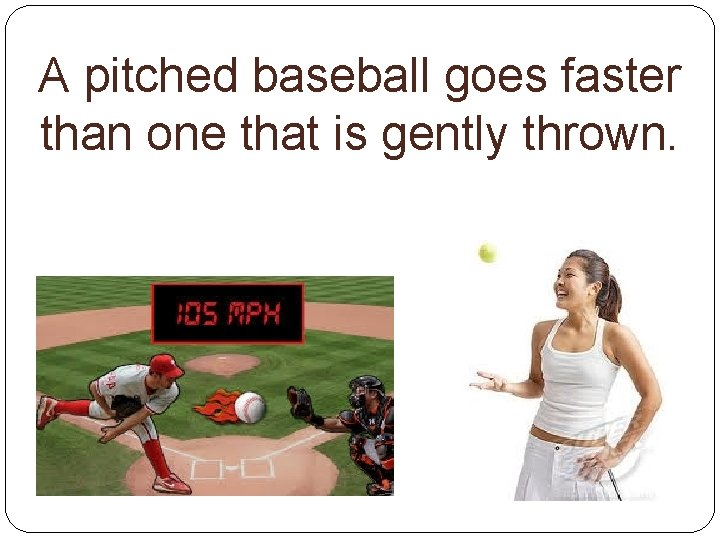A pitched baseball goes faster than one that is gently thrown.A swimmer pushes water back with his arms, but his body moves forward.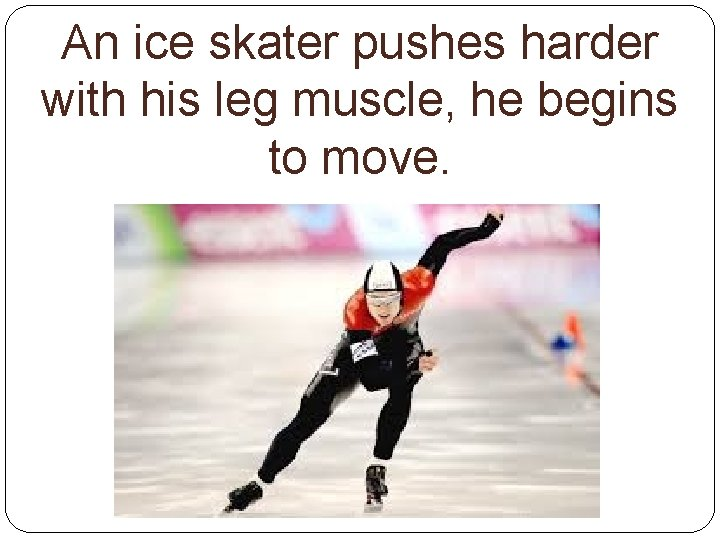An ice skater pushes harder with his leg muscle, he begins to move.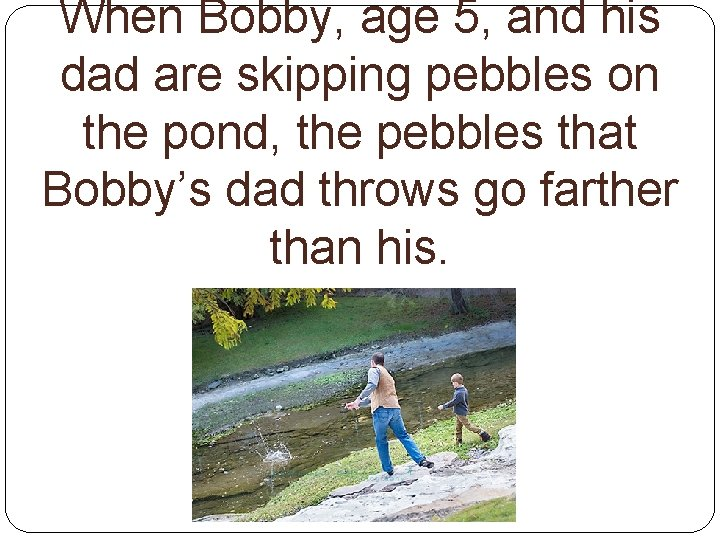When Bobby, age 5, and his dad are skipping pebbles on the pond, the pebbles that Bobby’s dad throws go farther than his.When you paddle a canoe, the canoe goes forward.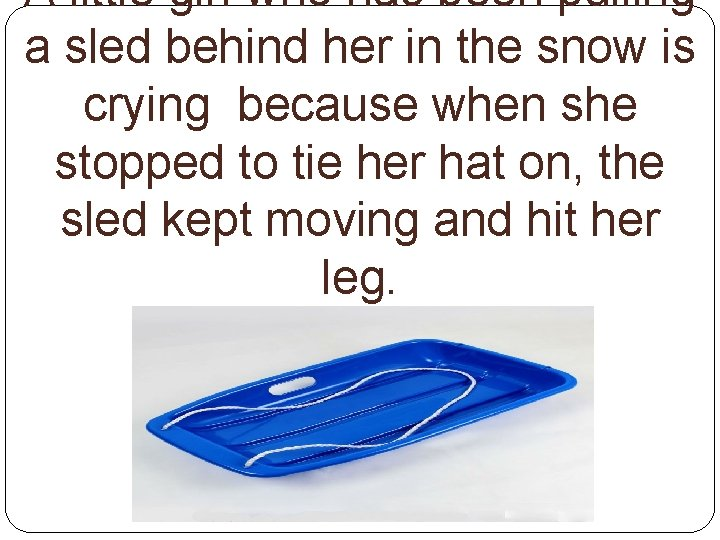A little girl who has been pulling a sled behind her in the snow is crying because when she stopped to tie her hat on, the sled kept moving and hit her leg.